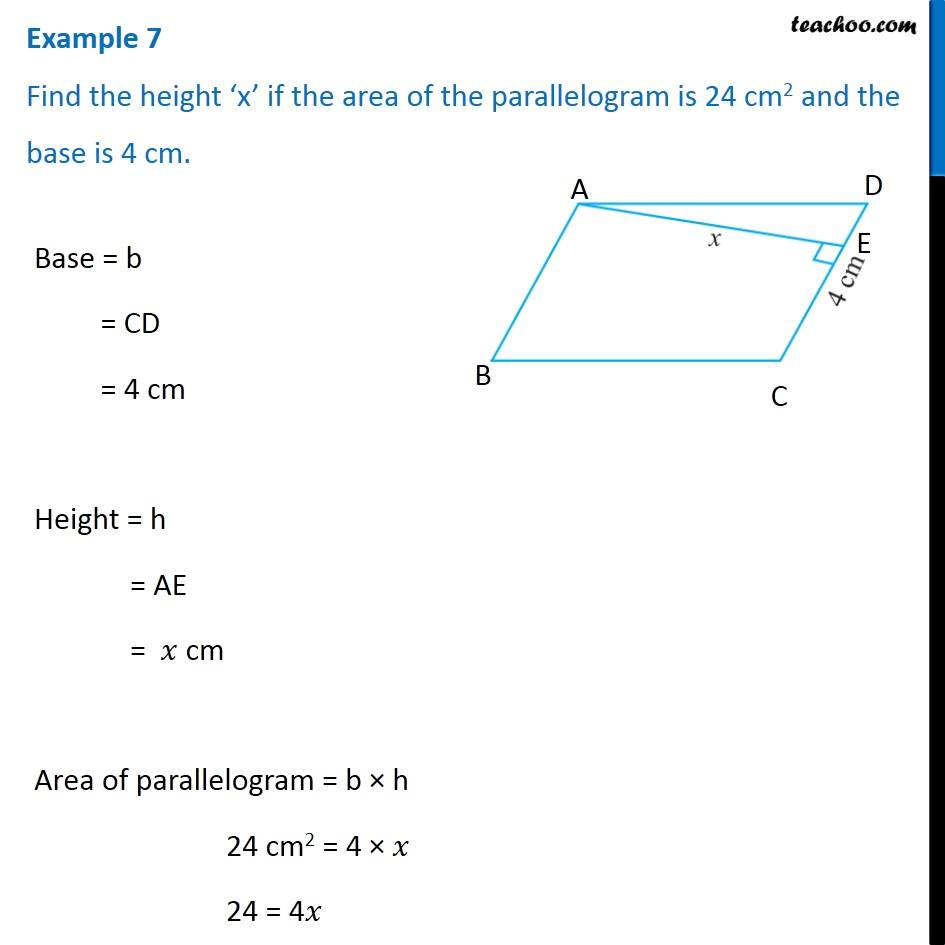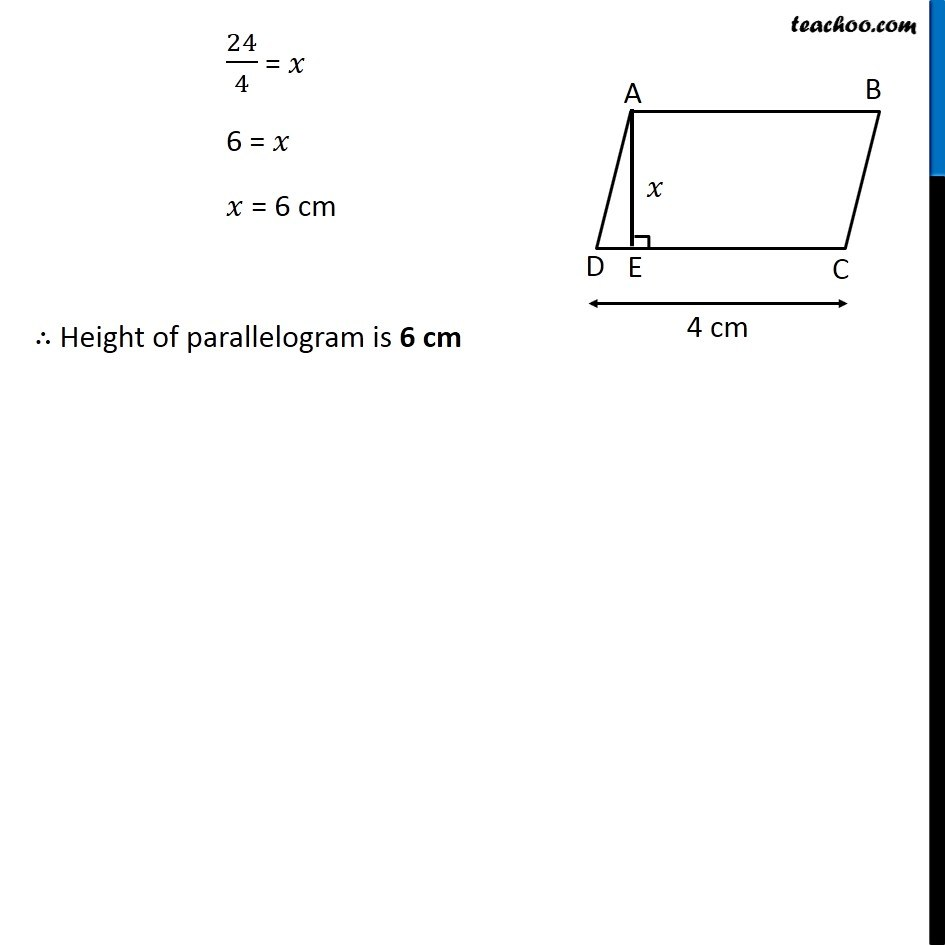Area of parallelogram

Chapter 9 Class 7 Perimeter and Area
Concept wiseGet live Maths 1-on-1 Classs - Class 6 to 12

### Transcript

Example 2 Find the height ‘x’ if the area of the parallelogram is 24 cm2 and the base is 4 cm. Base = b = CD = 4 cm Height = h = AE = 𝑥 cm Area of parallelogram = b × h 24 cm2 = 4 × 𝑥 24 = 4𝑥 24/4 = 𝑥 6 = 𝑥 𝑥 = 6 cm ∴ Height of parallelogram is 6 cm